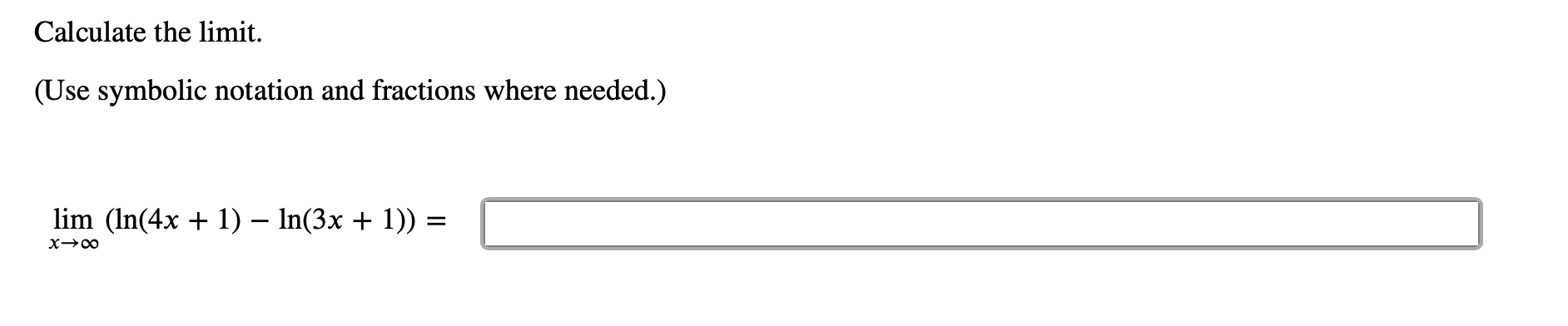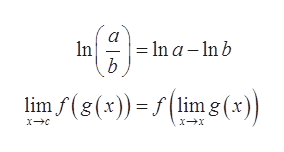Calculate the limit.(Use symbolic notation and fractions where needed.lim (ln(4x ) - In(3x 1))xOo

Questionhelp_outlineImage TranscriptioncloseCalculate the limit. (Use symbolic notation and fractions where needed. lim (ln(4x ) - In(3x 1)) xOo fullscreen
Step 1

To evaluate the limit of the function.

Step 2

Given:

Step 3

Formulae Used:

...help_outlineImage Transcriptionclose-In a- ln b n b limf(g(x))(lim g(x) fullscreen

Want to see the full answer?

See Solution

Want to see this answer and more?

Our solutions are written by experts, many with advanced degrees, and available 24/7

See Solution
Tagged in

Calculus